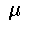The Ohio State University

DEPARTMENT OF MATHEMATICS

announces the
to be given byJeff Cheeger
Courant Institute of Mathematical Sciences
New York University

on the topic

Differentiability of Lipschitz Functions
on Metric Measure Spaces

 Tue. October 20 4:30 p.m. EA160 Wed. October 21 4:30 p.m. SM1153 Thurs. October 22 4:30 p.m. EA160

Room EA160 is in the Math Annex, 209 West 18th Avenue,
and SM1153 is in Smith Laboratory, 174 W. 18th Avenue.

## Abstract

A fundamental theorem of Rademacher asserts that Lipschitz functions f: Rn -->R, are differentiable almost everywhere. In our lectures, we will describe an extension of Rademacher's theorem to a certain class of metric measure spaces, (Z,). As a consequence of this extension, much of that part of calculus which concerns first derivatives (although not, of neccessity, the implicit function theorem) generalizes to spaces in this class. In various natural examples, for instance, the boundaries at infinity of 2-dimensional hyperbolic buildings, the measure, is Hausdorff measure and the Hausdorff dimension exceeds the topological dimension. In particular, the differentiability of Lipschitz functions (suitably interpreted) does not imply the existence of points at which the underlying space has a linear structure at the infinitesimal level.

Lecture 1. Overview and basic concepts
Lecture 2. Doubling measures, Poincaré inequalities and differentials
Lecture 3. Length spaces and a generalization of Rademacher's theorem